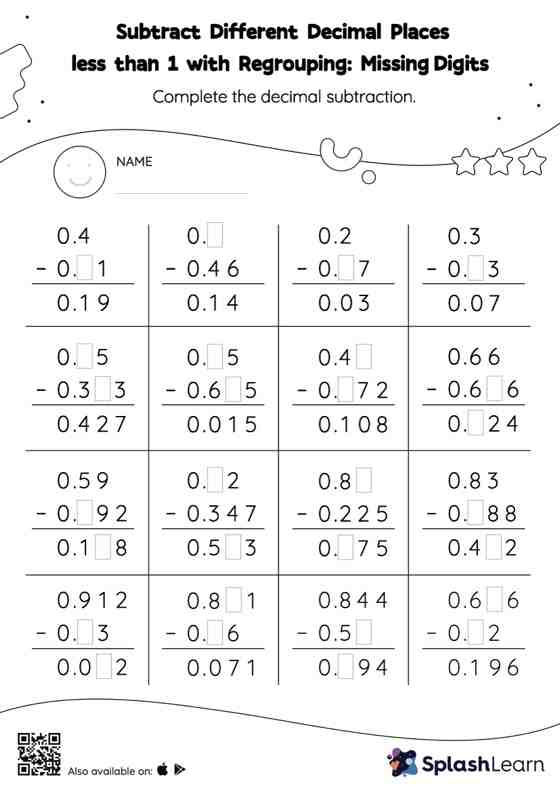# Subtract Different Decimal Places less than 1 with Regrouping: Missing Digits Worksheet

Home > Subtract Different Decimal Places less than 1 with Regrouping: Missing DigitsThis worksheet consists of a set of questions on subtraction where students are required to subtract different decimal places less than 1 with regrouping. Students find the missing number while subtracting decimals by using the relationship between addition and subtraction. To reach the answer, they also use the relationship between ones, tenths, hundredths, thousandths, etc., To regroup the numbers in subtract different decimal places less than 1 with regrouping worksheet.